Monday, January 21, 2019

How To Operate a Total Station - Steps - All components explained

Hi,

Total Station(TS) is a surveying instrument used to find the direction angles and distances of various lines. In this post, you will learn how to operate a Total Station. But first, let's know the components of a TS.

Components.
These images show the components of TS. The carrying handle can be fixed using to handle tightening screws. The sighting collimator is used for the rough sight of the prism. The Eyepiece is used for sighting the prism. Together with the eyepiece is telescope holding grip which is used to transit the telescope.All these are shown in the image below. Telescope holding grip is used for transiting the telescope, and telescope focusing knob is used to clear the image on the diaphragm.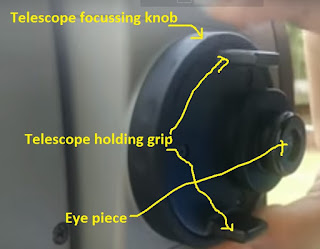Then there is a vertical tangent screw used for the small vertical movement of the TS so as to get the clear and straight line of sight so that the crosshairs intersect the prism. Also, there is a vertical motion clamp used to fix/lock the position of the vertical tangent screw.Similarly, there is a horizontal tangent screw and a horizontal motion clamp, which is used to control the horizontal rotation of the TS.
There are two display units, and the keys adjacent to them. The optical plummet screw is used to sight the ground and is used to level the TS.There are three levelling screws in the tri-bach which is fixed using the tri-bach fixing lever.When you power on the Display unit, it gets into its default mode - i.e.angle measurement mode. Display unit uses dot matrix LCD, which has four lines(rows) and 20 characters per line. The upper three lines display the measured data, and bottom line displays the softkeys' functions which change with the measuring mode.The image shown above shows all the keys with their respective functions.

Now before we take any measurements, it is important to do the centering of the TS and the prism. It is done as explained below.

Step.1 Level the Total Station(TS)

Put the Total Station on the Tripod Stand. The approximately level the lower bubble by arranging the three legs of the tripod stand.Then note that there is a horizontal bubble tube, in which the bubble will not be at centre if the total station is not levelled.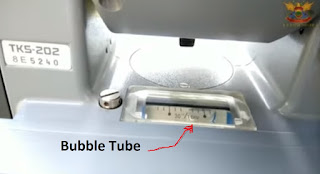Bring this bubble tube parallel with the imaginary line joining two of the three screws. And then rotate both of them together either inwards or outwards. Note the movement of the bubble and rotate the screws until the bubble comes to the centre.

Now rotate the total station such that the bubble tube is perpendicular to that imaginary line between the two screws. When you do so, the bubble may move from the central position, so now rotate the third screw until it again comes to the centre. Now bring the tube to the initial position and again check the bubble and repeat the process until the bubble is at the centre in both of the positions.
Now your Total Station is Levelled.

Step.2 Put the Stand and Prism at the station of interest and Level it

There is a stand and a prism which is sighted from the TS. Fix the prism on the stand, and note that there a bubble on the stand too, so you have to level the stand too.Simply fix the vertical position of the stand until the bubble is centred.  Now sight the TS through the sighting collimator.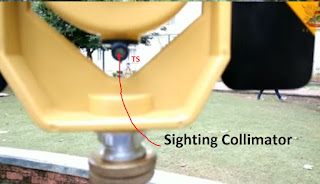Now that centring is done, let's take the measurements to find the horizontal distance between two points.

Horizontal Distances, slope distance, co-ordinates and angle Measurement using Total Station.

So here we have two points, one - where the instrument is set up and the other where the prism is. Use the sighting collimator to do the approximate bisection of the prism.
Then sight through the eyepiece and use the focussing screw to bisect the prism with the crosshairs. Then tighten up the vertical tangent screw using the vertical tangent clamp.
Use the eyepiece to get a clear prism image. Once it is done, click on the distance measurement mode key.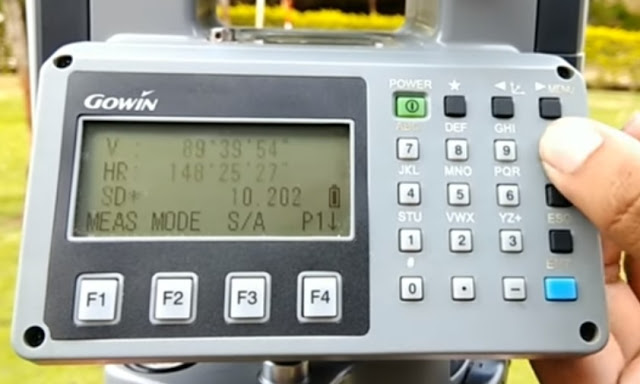Display Unit of Total station showing the Slope Distance(SD)

Similarly, when you click the co-ordinate key on, it will show the coordinates (x,y,z) of the station. And when it is in angle measurement mode, it will display horizontal angle with the right face(HR) and vertical angle(V.)

Thanks!

Monday, August 6, 2018

Sand Cone Method to Determine Density - A solved Example

Hi,
This problem deals with the Sand Cone Test used to determine the density of the compacted soil.

Problem: A sand cone density test was conducted on soil compaction at a land development project. It has the following data:
the volume of the standard Proctor mould = 1/30 cubic feet
Mass of the sand drained to fill the mould = 3.30 lbs
Mass of the sand to fill the cone = 0.53 lbs
Mass of the soil dug from the hole = 8.11 lbs (and have water content w = 11.11%)
Mass of jug + Sand + Cone before used to fill the hole = 10.20 lbs
Mass of jug+ Sand cone after used = 3.20 lbs

Determine: (A) The density of the Ottawa Sand(pcf)
(B) The volume of the cone (cubic feet)
(C) The volume of the hole dug  + Cone (Cubic ft)
(D)  The volume of the hole dug
(E)  The dry density of the compacted soil

Solution (see image):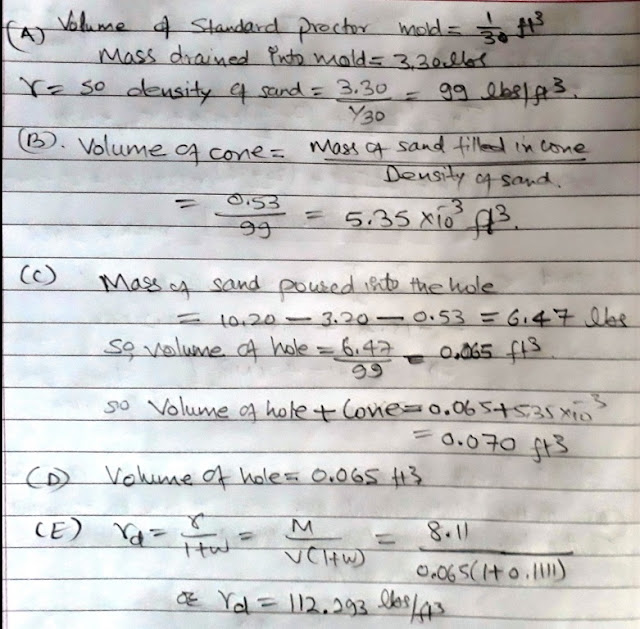Thanks!

Monday, July 30, 2018

Treating the Dredged Marine Sediment with Bentonite and OPC

Hi
Sediments that are dredged from the water bodies such as reservoir, lakes or oceans are weak in strength and highly compressible, in general. This is nothing more than a waste material. However, it can be treated with certain chemicals such as the Bentonite and OPC(Ordinary Portland Cement) to give it the desired properties of a fill material in back-fills.It is found that the OPC acts as the binder and provides the strength, while the Bentonite provides the volume and also the strength to the clay. Therefore this treated soil can then be used as the fill, or also it can be used to form the dikes or trenches above the water surface.

Thanks!

Tuesday, July 10, 2018

Solved Example of Pinned Truss - the average normal and shear stresses in members and pins respectively and Pin Diamater

Hi,
A simple truss has pin-connected members. The members carry only axial forces, but the pins have to bear the shear stresses. Given example shows the calculation for the minimum required diameter of pin connected in double shear.

Problem: Two structural members AB and BC (shown below) are connected by a pin at B that is subjected to two 5-kip horizontal forces. The pins used at A and C are identical to the pin at B. The allowable shear stress for pins is 15 ksi, and the allowable bearing stress for members AB and BC is 20 ksi. Determine:
(a) the maximum average normal stress in members AB and BC.
(b) the minimum required diameters of the pins.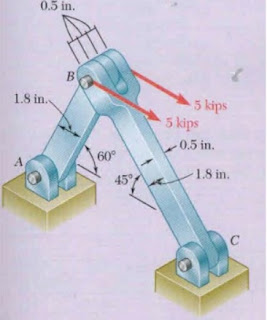Solution:Area of traverse by Average End Area Method - Solved Example

Hi,

For the trapezoidal sections, when the end heights are given, the area can be found by averaging the end heights and multiplying it with the distance between those heights.

Given examples show the closed traverses with the straight baselines.Solution:Friday, April 20, 2018

How to Balance a Closed Traverse - Use of Compass(Bowditch) Rule - Solved Example

Hi,

Problem:
Following is the Field Data for a 4 sided, closed traverse, and you have to find the azimuth, bearings and corrected distances using Compass Rule or Bowditch Method.Solution:
Download the image, solution is written for the angular closure, correction, azimuth, bearings, latitude and departure and corrected distance calculations.Thursday, April 19, 2018

Derivation of Mass moment of Inertia for a Solid Cone

Hi,

Problem:
Derive the Mass Moment of Inertia of a solid cone with given mass density and angle half at vertex equal to 35 degrees. If the length of the cone is 5 m and mass density is 2.5 kg/m3, what is the mass moment of inertia.

Solution:How To Operate a Total Station - Steps - All components explained

Hi, Total Station(TS) is a surveying instrument used to find the direction angles and distances of various lines. In this post, you will ...